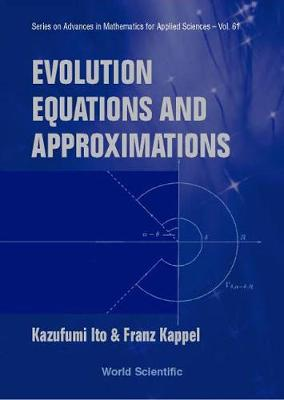•Evolution Equations And Approximations - Series on Advances in Mathematics for Applied Sciences 61 (Hardback)

(author), (author)
£133.00
Hardback 520 Pages / Published: 28/05/2002
• We can order this

Usually dispatched within 3 weeks

This book presents an approximation theory for a general class of nonlinear evolution equations in Banach spaces and the semigroup theory, including the linear (Hille-Yosida), nonlinear (Crandall-Liggett) and time-dependent (Crandall-Pazy) theorems.The implicit finite difference method of Euler is shown to generate a sequence convergent to the unique integral solution of evolution equations of the maximal monotone type. Moreover, the Chernoff theory provides a sufficient condition for consistent and stable time integration of time-dependent nonlinear equations. The Trotter-Kato theorem and the Lie-Trotter type product formula give a mathematical framework for the convergence analysis of numerical approximations of solutions to a general class of partial differential equations. This book contains examples demonstrating the applicability of the generation as well as the approximation theory.In addition, the Kobayashi-Oharu approach of locally quasi-dissipative operators is discussed for homogeneous as well as nonhomogeneous equations. Applications to the delay differential equations, Navier-Stokes equation and scalar conservation equation are given.

Publisher: World Scientific Publishing Co Pte Ltd
ISBN: 9789812380265
Number of pages: 520
Weight: 871 g
Dimensions: 198 x 126 x 32 mm